# DPL 9 Help

Binomial Distribution

#Binomial Distribution

The Binomial Distribution appears whenever assumptions of independent, 2-outcome trials with stable probabilities are used. It is used to represent the number of successes in a given number of Bernoulli trials (a Bernoulli trial has two outcomes with fixed probabilities). The Binomial Distribution has been used in analytical studies for casinos, and also frequently with mortality data. In failure analysis it is a discrete distribution representing the number of failures in a given number of trials, assuming a constant probability of failure in each trial.

The Binomial Distribution represents the probability of getting k heads in n flips of a biased coin. The probability of a head on any flip is p. The parameters of the distribution are n, which must be a positive integer, and p. The distribution is: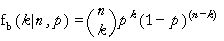where k=0,1,...,n, n=1,2,..., and 0<p<1. Also, n must be greater than the number of points in the approximation.

The equations for the mean and variance are: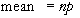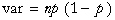The equations for the parameters are: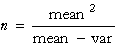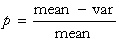The mean must be greater than the variance. Also the expression for n must produce a positive integer.

Versions: DPL Professional, DPL Enterprise, DPL Portfolio